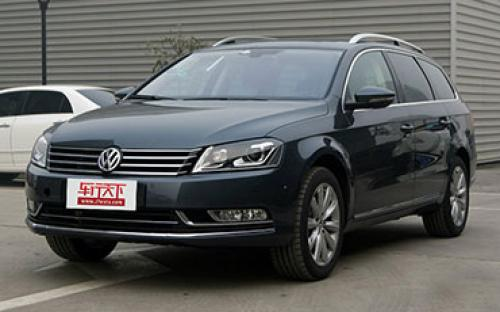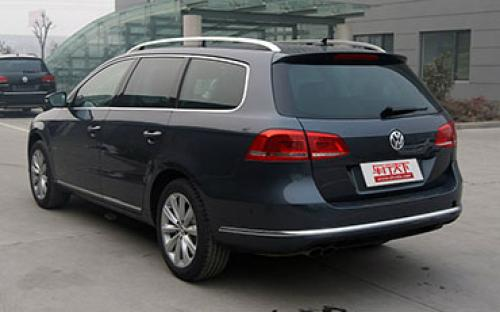## 大众(进口) 迈腾(进口)12 种颜色可选2012款最低售价：34.28 万元起

4771(mm)1820(mm)1516(mm)##### 配置亮点：
• 胎压监测装置

• ISOFIX儿童座椅接口

• 车身稳定控制(ESC/ESP/DSC等)

• 电动天窗

• 定速巡航

• 后倒车雷达

• 真皮座椅

• GPS导航系统

• 氙气大灯

• 后视镜加热大众(进口) 迈腾(进口) 在售车型

排量 车型 厂商指导价 本地最低报价 购车工具
2.0T 旅行版 2.0TSI 舒适型 6挡双离合 34.28万 34.28万

询底价+对比
旅行版 2.0TSI 豪华型 6挡双离合 39.15万 39.15万

询底价+对比
旅行版 2.0TSI 四驱舒适型 6挡双离合 36.52万 36.52万

询底价+对比
旅行版 2.0TSI 四驱豪华型 6挡双离合 41.60万 41.60万

询底价+对比

大众(进口) 迈腾(进口) 经销商

查看更多 >>
更多 >>

本地降价排名

### 大众(进口) 迈腾(进口) 动力加速

迈腾(进口) 0-100公里加速时间分布在 7.7-7.8秒 属于 运动级

动力级别 加速时间 车型
运动级(2款) 7.7s 旅行版 2.0TSI 舒适型旅行版 2.0TSI 豪华型
7.8s 旅行版 2.0TSI 四驱舒适型旅行版 2.0TSI 四驱豪华型

大众(进口) 迈腾(进口) 视频

大众(进口) 迈腾(进口) 新闻资讯

文化 超过0次关注

# 大众8座工厂投产 在中国推广MQB生产线

技术 超过0次关注

近日，有媒体报道称，大众未来将重点在中国推广MQB平台新车并布置产能，近期内在华MQB工厂将达到8座。

# 迈腾旅行版静态体验 真正的“家”用车

评测 超过6734次关注

旅行车应该说是今年广州车展的一大主角，除了刚刚上市的宝马5系旅行版之外，在大众展台上亮相的全新迈腾旅行版也是值得我们关注的一个焦点。在全新一代一汽大众迈腾...

# 实拍大众迈腾Alltrack 旅行是一种态度

导购 超过4866次关注

在去年的广州车展上，包括宝马、大众、沃尔沃在内的多个厂商纷纷展示自己的旅行车产品，让人不禁以为旅行车在国内将迎来春天。而作为最早把旅行车引入到中国市场的...

# 大众40余款车型价格上调 最高涨2.84万

新闻 超过0次关注

近日我们从大众中国了解到，它们上调了一部分进口车型的市场指导价格，涉及包括甲壳虫、迈腾旅行版、途锐、夏朗以及Tiguan等车型，最高涨幅也达到了2.84万元。

# 进口大众多款车搭载车联网系统 4年免费

新闻 超过4862次关注

大众进口汽车正式推出了车联网服务。该服务将搭载于进口Tiguan、甲壳虫、尚酷、高尔夫R敞篷轿车、夏朗、迈腾旅行轿车、迈腾四驱旅行车等多款大众进口车型上，并为用...

猜你喜欢

﻿
• 快速找车
• 选择品牌
• 选择品牌
• A  奥迪
• A  阿斯顿·马丁
• A  阿尔法·罗密欧
• B  宝沃
• B  布加迪
• B  巴博斯
• B  保时捷
• B  宾利
• B  奔驰
• B  宝马
• B  本田
• B  别克
• B  标致
• B  比亚迪
• B  宝骏
• B  北汽制造
• B  北汽新能源
• B  北汽幻速
• B  北汽威旺
• B  北京汽车
• B  奔腾
• B  北汽绅宝
• C  长安
• C  长安商用
• C  长城
• C  昌河
• D  大众
• D  道奇
• D  DS
• D  东南
• D  东风风神
• D  东风风行
• D  东风小康
• D  东风风度
• D  东风
• F  福特
• F  丰田
• F  菲亚特
• F  法拉利
• F  福田
• F  福迪
• F  福汽启腾
• G  观致
• G  广汽传祺
• G  广汽吉奥
• G  GMC
• H  红旗
• H  汉腾汽车
• H  哈弗
• H  哈飞
• H  海格
• H  海马
• H  华颂
• H  黄海
• H  华泰
• H  恒天
• J  吉利汽车
• J  捷豹
• J  Jeep
• J  江淮
• J  江铃
• J  金杯
• J  九龙
• J  金旅
• K  凯翼
• K  凯迪拉克
• K  克莱斯勒
• K  科尼塞克
• K  卡威
• K  开瑞
• L  路虎
• L  林肯
• L  劳斯莱斯
• L  兰博基尼
• L  雷克萨斯
• L  铃木
• L  雷诺
• L  理念
• L  力帆
• L  莲花汽车
• L  猎豹
• L  路特斯
• L  陆风
• M  马自达
• M  MG
• M  MINI
• M  玛莎拉蒂
• M  摩根
• M  迈凯轮
• N  纳智捷
• O  欧宝
• O  讴歌
• O  欧朗
• Q  奇瑞
• Q  起亚
• Q  启辰
• R  日产
• R  荣威
• R  瑞麒
• S  三菱
• S  斯威汽车
• S  萨博
• S  smart
• S  斯柯达
• S  斯巴鲁
• S  思铭
• S  双龙
• S  上汽大通
• S  双环
• T  特斯拉
• T  腾势
• W  沃尔沃
• W  五菱汽车
• W  五十铃
• W  威兹曼
• W  威麟
• X  现代
• X  雪佛兰
• X  雪铁龙
• X  西雅特
• Y  一汽
• Y  英菲尼迪
• Y  英致
• Y  依维柯
• Y  野马汽车
• Y  永源
• Z  众泰
• Z  中华
• Z  中兴
• Z  知豆
• 选择车系
• 选择车系
• 车型对比
• 选择品牌
• 选择品牌
• A  奥迪
• A  阿斯顿·马丁
• A  阿尔法·罗密欧
• B  宝沃
• B  布加迪
• B  巴博斯
• B  保时捷
• B  宾利
• B  奔驰
• B  宝马
• B  本田
• B  别克
• B  标致
• B  比亚迪
• B  宝骏
• B  北汽制造
• B  北汽新能源
• B  北汽幻速
• B  北汽威旺
• B  北京汽车
• B  奔腾
• B  北汽绅宝
• C  长安
• C  长安商用
• C  长城
• C  昌河
• D  大众
• D  道奇
• D  DS
• D  东南
• D  东风风神
• D  东风风行
• D  东风小康
• D  东风风度
• D  东风
• F  福特
• F  丰田
• F  菲亚特
• F  法拉利
• F  福田
• F  福迪
• F  福汽启腾
• G  观致
• G  广汽传祺
• G  广汽吉奥
• G  GMC
• H  红旗
• H  汉腾汽车
• H  哈弗
• H  哈飞
• H  海格
• H  海马
• H  华颂
• H  黄海
• H  华泰
• H  恒天
• J  吉利汽车
• J  捷豹
• J  Jeep
• J  江淮
• J  江铃
• J  金杯
• J  九龙
• J  金旅
• K  凯翼
• K  凯迪拉克
• K  克莱斯勒
• K  科尼塞克
• K  卡威
• K  开瑞
• L  路虎
• L  林肯
• L  劳斯莱斯
• L  兰博基尼
• L  雷克萨斯
• L  铃木
• L  雷诺
• L  理念
• L  力帆
• L  莲花汽车
• L  猎豹
• L  路特斯
• L  陆风
• M  马自达
• M  MG
• M  MINI
• M  玛莎拉蒂
• M  摩根
• M  迈凯轮
• N  纳智捷
• O  欧宝
• O  讴歌
• O  欧朗
• Q  奇瑞
• Q  起亚
• Q  启辰
• R  日产
• R  荣威
• R  瑞麒
• S  三菱
• S  斯威汽车
• S  萨博
• S  smart
• S  斯柯达
• S  斯巴鲁
• S  思铭
• S  双龙
• S  上汽大通
• S  双环
• T  特斯拉
• T  腾势
• W  沃尔沃
• W  五菱汽车
• W  五十铃
• W  威兹曼
• W  威麟
• X  现代
• X  雪佛兰
• X  雪铁龙
• X  西雅特
• Y  一汽
• Y  英菲尼迪
• Y  英致
• Y  依维柯
• Y  野马汽车
• Y  永源
• Z  众泰
• Z  中华
• Z  中兴
• Z  知豆
• 选择车系
• 选择车系
• 选择车型
• 选择车型
• 意见反馈